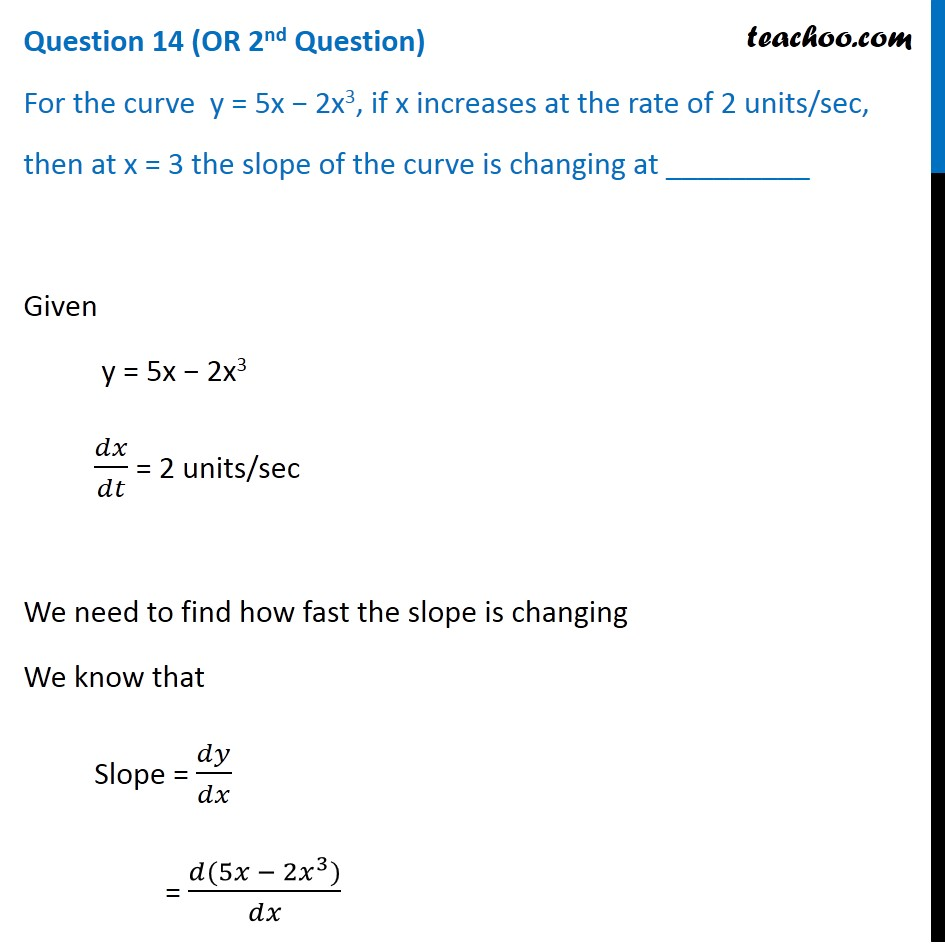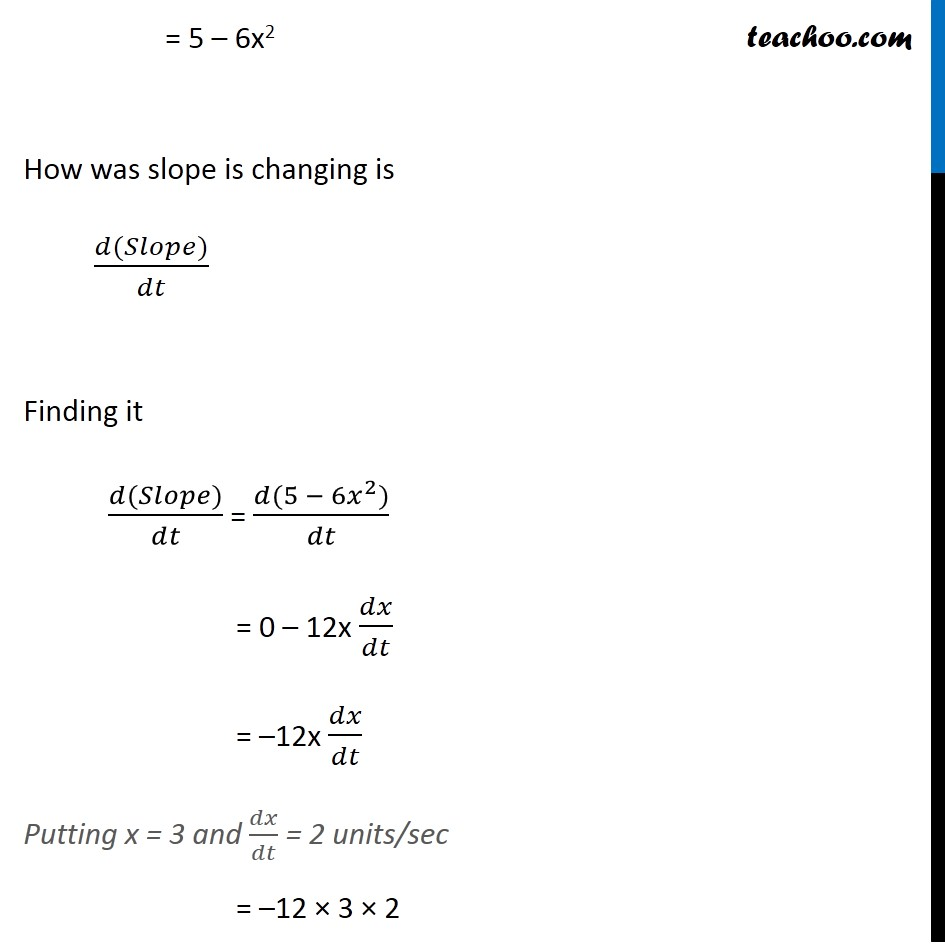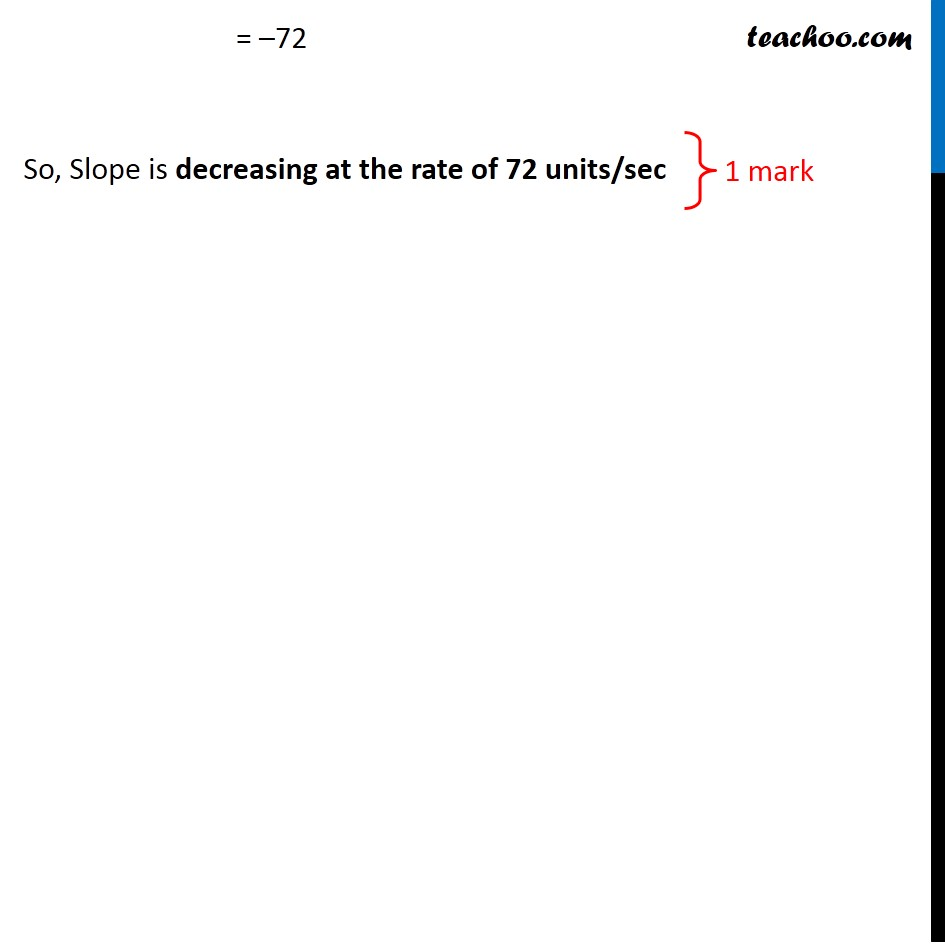CBSE Class 12 Sample Paper for 2020 Boards

Class 12
Solutions of Sample Papers and Past Year Papers - for Class 12 Boards

## For the curve  y = 5x − 2x 3 , if x increases at the rate of 2 units/sec, then at x = 3 the slope of the curve is changing at _________Get live Maths 1-on-1 Classs - Class 6 to 12

### Transcript

Question 14 (OR 2nd Question) For the curve y = 5x − 2x3, if x increases at the rate of 2 units/sec, then at x = 3 the slope of the curve is changing at _________ Given y = 5x − 2x3 𝑑𝑥/𝑑𝑡 = 2 units/sec We need to find how fast the slope is changing We know that Slope = 𝑑𝑦/𝑑𝑥 = (𝑑(5𝑥 − 2𝑥^3))/𝑑𝑥 = 5 – 6x2 How was slope is changing is (𝑑(𝑆𝑙𝑜𝑝𝑒))/𝑑𝑡 Finding it (𝑑(𝑆𝑙𝑜𝑝𝑒))/𝑑𝑡 = (𝑑(5 − 6𝑥^2))/𝑑𝑡 = 0 – 12x 𝑑𝑥/𝑑𝑡 = –12x 𝑑𝑥/𝑑𝑡 Putting x = 3 and 𝑑𝑥/𝑑𝑡 = 2 units/sec = –12 × 3 × 2 = –72 So, Slope is decreasing at the rate of 72 units/sec